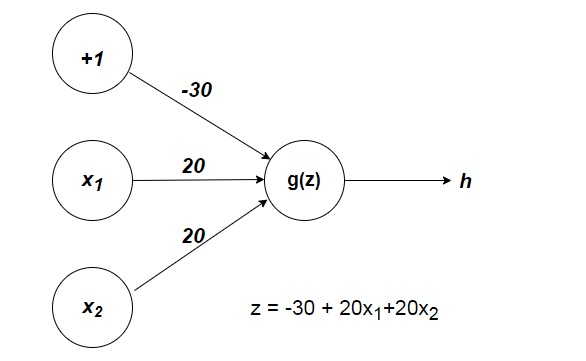### A Gentle Introduction to Neural Networks for Machine Learning

In this post we discuss the basics of the artificial neural network- what is non-linear classification, analogy between the neurons and brains and defining a simple artificial neural network. This is the fourth post in the series of machine learning. I suggest to read the previous posts related to machine learning.

### Non Linear Classification

When our training data is very complex then performing linear regression is not best. The training data is not best fitted with linear hypothesis, we include some terms like quadratic, cubic or even higher powers. These terms will make the hypothesis nonlinear. Suppose we have three features x1, x2, x3 and want to add all quadratic terms to make the hypothesis nonlinear, we have to add squares of these three terms and products of these taking two at a time. So in this way it will make six features. If our training set has more features say 100, then to make a quadratic hypothesis function we have more than five thousands of features.

### Neurons and Brain

The learning through neural networks is like we human being learn. The algorithms in neural network tries to mimic brain. Dendrites and axons are two important parts in neuron in the brain for input and output respectively.

### A Simple Neural Network

A simplest neural network may have two layers, first layer (input nodes) and the final layer (output nodes). For a more complex neural network we have one or more layers often called the hidden layers (hidden nodes) in between the input and output layers. The parameters, whatever we are using so for, are called weights in neural network model. The first layer nodes are connected to the second layer nodes and so for.

Generally used hypothesis function is sigmoid (logistic) function (Fig 2) often called activation function. The hidden nodes are often called the activation units or activation nodes. A set of parameters (weights) are applied on the input to obtain one activation node, other set of weights are applied to find other activation node. The hypothesis output is the logistic function applied on the sum of the activation nodes multiplied by other set of parameters.

In the vectorized implementation of the activation function or hypothesis function. we define a variable z, which is equal to the product of two matrices namely the features matrix or the activation matrix and the parameter matrix. At final layer we apply logistic function g on z to get the hypothesis output, which can be given in a vector form as h(x) = g(z). Other way

h(x)=a(j+1)=g(z(j+1)) where j represents the layer from which input is taken and output is calculated for j+1 layer.

A simple example of neural network to predict x1 AND x2, which holds true only when both x1 and x2 are 1. Here we have x0=1, it is called a bias variable. we have parameters matrix [-20,10,10]. so z = -20+10x1+10x2

h(x)=g(−20+20x1+20x2).

we have all combination of the 0 and 1 for the x1 and x2 we find that when both the variables are 1 then the h(x) is 1.

You can create a simple neural network representation of AND operation and below:Fig 1: A simple neural network to perform AND operationFig 2: Sigmoid Function

Here we have created a simple neural network to perform AND operation without using AND gates. Similarly we can create simple neural networks to perform other gate operations.

Multiclass Classification- in multiclass classification the hypothesis function returns a vector of values this allows us to classify the data into multiple class. The final layer of nodes are multiplied by the parameters matrix this gives us a vector, on this vector the logistic function is applied to get the vector of hypothesis values.